# GMAT Math : Calculating percents

## Example Questions

← Previous 1 3 4 5 6 7

### Example Question #1 : Percents

330 is what percent of 11?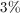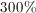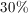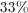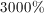Explanation:

This problem can be solved by the equation: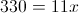, whereis the answer in terms of a percentage. To solve forboth sides are divided by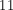: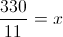. This can be simplified to: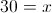. Now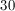is converted to a percentage to find the answer.as a percentage is### Example Question #2 : Percents

Three years ago, Anum invested $25,000 in a new mutual fund account. The value of the account increased by 15% during the first year, increased by 7% during the second year, and decreased by 15% during the third year. What is the approximate value of the account today? Possible Answers: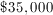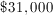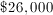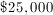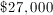Correct answer:Explanation: The first year increase of 15% can be represented as 1.15; the second year increase of 7% can be represented as 1.07; and the third year decrease of 15% can be represented as 0.85. Multiply the original investment by each annual change. 25,000(1.15)(1.07)(0.85) = 26,148 approx.$26,000

### Example Question #3 : Percents

Max the cat has 70 toy mice. If 30% of the mice are new, how many mice are not new?

60

40

30

49

21

49

Explanation:

If 30% of the mice are new, then 70% are not new.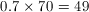### Example Question #1 : Percents

37% of the greatest common factor of 50 and 350 is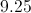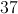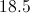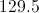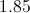Explanation:

The greatest common factor of 50 and 350 is simply 50.  Now we must take 37% of 50.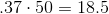.

A shirt costs $60. The price was then increased by 30%. What is the new price as expressed in terms of the original price? Possible Answers: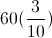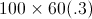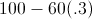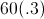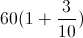Correct answer:Explanation: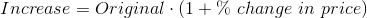### Example Question #6 : Percents A GMAT score increases from 450 to 540. By what percent did the score increase? Possible Answers: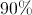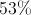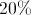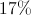Correct answer:Explanation: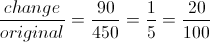### Example Question #7 : Percents Out of 60 students, 15 prefer to study at night. What percent of students prefer nighttime studying? Possible Answers: 60% 15% 4% 25% Correct answer: 25% Explanation: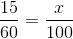Cross multiply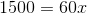Divide both sides by 60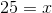### Example Question #8 : Percents Geri earns a commission of 22.5% on everything she sells for KM Cosmetics. As of the fifteenth of the month, she has sold$2,100 worth of cosmetics. How much more in cosmetics must she sell in order to earn at least $900 commission for the month? Possible Answers: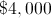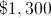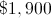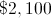She has already sold enough to earn$900 commission.Explanation:

Commission can be calculated by muliplying the commission rate - 22.5%, or 0.225 - by the amount of sales, so the amount of sales needed can be found by dividing the desired commission by 0.225.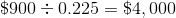Since Geri has already sold \$2,100 worth of cosmetics, she needs to sell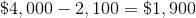more.

### Example Question #1 : Calculating Percents

A number is multiplied by six; then its decimal point is moved to the left three spaces. This is the same as taking what percent of the number?%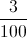%%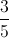%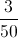%%

Explanation:

Let's do these two things to 100. Multiplying 100 by 6 yields 600; moving the decimal point three places left changes 600, or 600.0, to 0.60.

This means that the new number is 0.60% , or%, of the original number.

### Example Question #4 : Calculating Percents

A number is divided by two; then its decimal point is moved to the left two spaces. This is the same as taking what percent of the number?%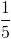%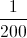%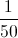%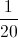%%
This means that the new number is 0.50% , or%, of the original number.